# POST

## 电容式触摸检测技术原理

ITO全称是纳米铟锡金属氧化物，主要有这两点特性，透明和导电。适合应用于在屏幕上贴合透明的导电层的需求。

ITO的图形常见的有菱形/三角形/长条形/毛毛虫形等。以及基于菱形的一些变形图案，比如雪花形等。其中菱形/长条形应用于两层ITO的设计，三角形应用于单层单点的触控，毛毛虫的图案用于单层多点的开发。性能方面也有不同，比如菱形的方案由于电场的分布一致性好，因此在触摸检测的精度和线性度方面有优势，另外变形的方案，比如雪花形，是在菱形的方案上增加了X,Y层的耦合面积，因此在灵敏度方面更好，另外对于一些小面积的物体，比如1mm的passive stylus的检测精度上有提升。

## 检测原理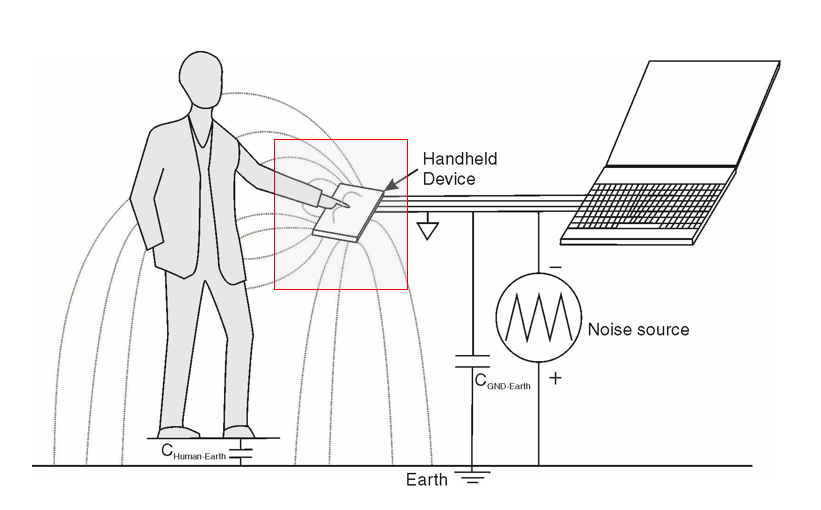### 电路模型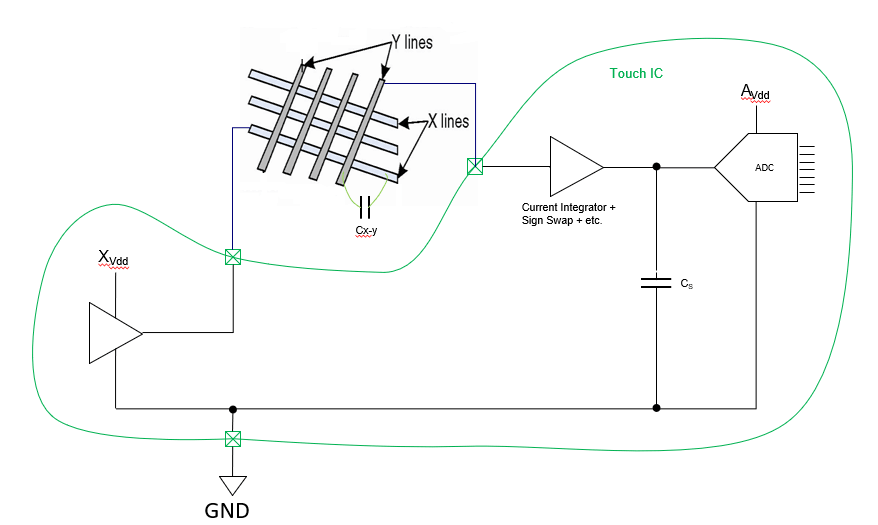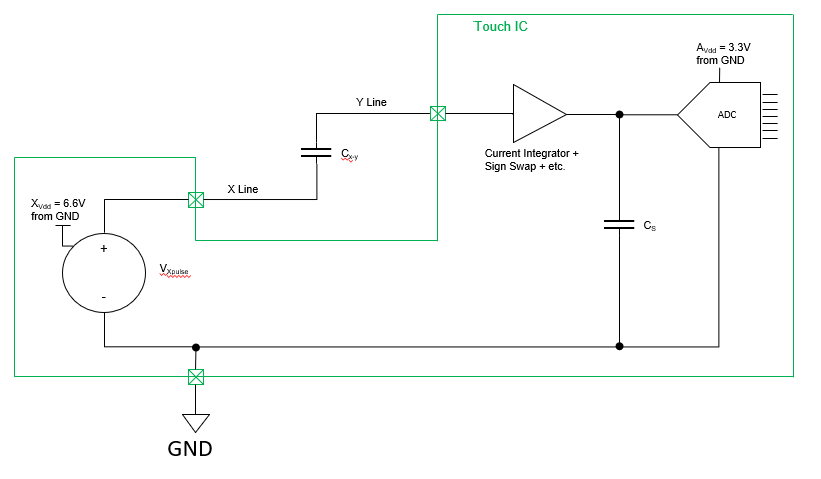Q = C × ΔV （Qtotal = Csystem × ΔV）

Csystem = 1/(1/Cx-y + 1/Cs) = 1/(1/2pF + 1/18pF) = 1.8pF

Qtotal = 1.8pF × 6.6V = 11.88pC

ΔVacross Cs = Q / Cs = 11.88pC / 18pF = 0.66V。

0.66V / 3.3V × 1024 = 205 ADC counts

11.88pc 0.66v 205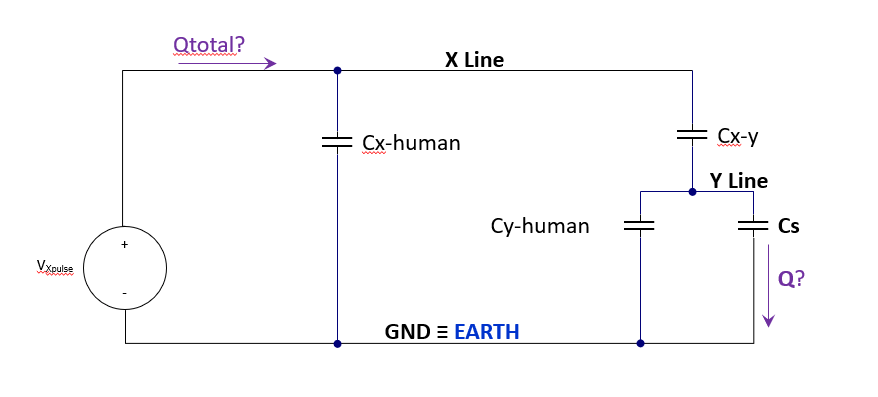Ca = Cy-human + Cs = 2pF + 18pF = 20pF

Cb = 1 / (1/Cx-y + 1/Ca) = 1 / (1/2pF + 1/20pF) = 1.81pF

Csystem = Cx-human + Cb = 2pF + 1.81pF = 3.81pF

Qtotal = Csystem × ΔV = 3.81pF × 6.6V = 25.2pC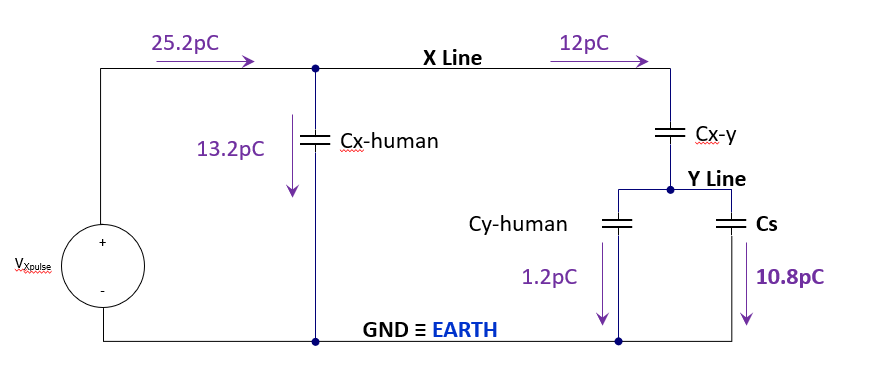ΔVacross Cs = Q / Cs = 10.8pC / 18pF = 0.6V

11.88pc 0.66v 205
10.8pc 0.6v 186